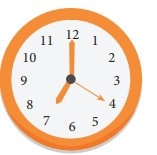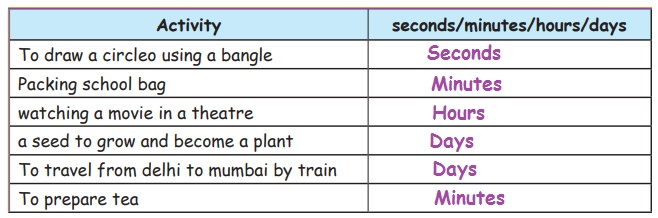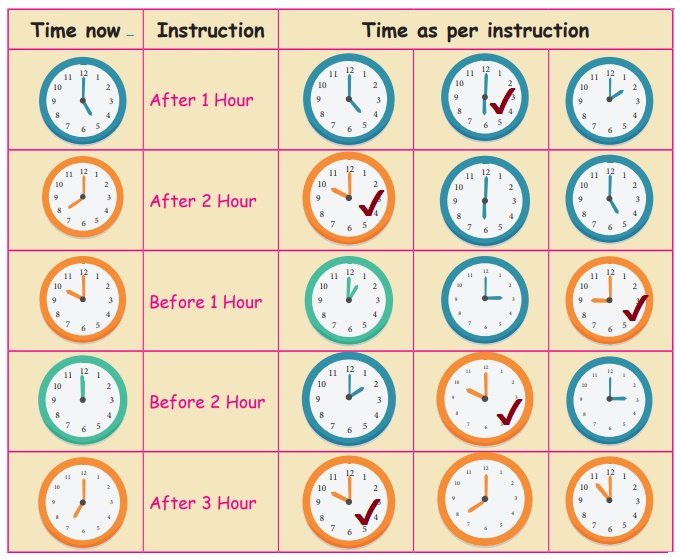Home | | Maths 3rd Std | Reading Time

## Chapter: 3rd Maths : Term 1 Unit 5 : Time

I am a clock. I have numbers from 1 to 12 marked on me. I have two hands, a short hand called the hour hand and a long hand called the minute hand. Some clocks have another hand called the seconds hand.I am a clock. I have numbers from 1 to 12 marked on me. I have two hands, a short hand called the hour hand and a long hand called the minute hand. Some clocks have another hand called the seconds hand.

The hour hand takes one hour to move from one number to the next number. The minute hand moves faster than the hour hand and takes five minutes to move from one number to the next number. The seconds hand moves very faster and it takes five seconds to move from one to the next number.

Let us draw the clock in 3 steps.Step:1 1,2,3,……59,60.

Step:2 skip count in 5’s as 5,10,15,20,…….55,60.

Step :3 Write 1,2,3,…..12 directlty below 5,10,15,….

12 hours + 12 hours = 24 hours = 1 day

Teacher’s note : Teacher can explain the hour hand, minute hand, and second hand by using clocks.

Practice

1. Read the Time and write in two ways. One is done for you.2. Draw hands on the clock’s face to show the time given below.3. Write the time taken to do the following activities (hours, minutes, seconds or days).Try this

In one minute how many times can you.

2. Skip a rope 70

3. Jump up and down 50

Let us know

60 seconds = 1 minutes

60 minutes = 1 hour

Activity 1

Tick the clock which shows the correct time as per the instruction.Tags : Time | Term 1 Chapter 5 | 3rd Maths , 3rd Maths : Term 1 Unit 5 : Time
Study Material, Lecturing Notes, Assignment, Reference, Wiki description explanation, brief detail
3rd Maths : Term 1 Unit 5 : Time : Reading Time | Time | Term 1 Chapter 5 | 3rd Maths